Linking Generative Models, Nearest Neighbor Retreival, and Data Augmentation
NeurIPS 2020 [pdf] [code]## Abstract

We introduce Exemplar VAE, a generalization of kernel density estimation using neural netowrks. Exemplar VAE is a variant of VAE with a non-parametric prior in the latent space based on a Parzen window estimator. To sample from it, one first draws a random exemplar from a training set, then stochastically transforms that exemplar into a latent code and a new observation. We propose retrieval augmented training (RAT) as a way to speed up Exemplar VAE training by using approximate nearest neighbor search in the latent space to define a lower bound on log marginal likelihood. To enhance generalization, model parameters are learned using exemplar leave-one-out and subsampling. Experiments demonstrate the effectiveness of Exemplar VAEs on density estimation and representation learning. Importantly, generative data augmentation using Exemplar VAEs on permutation invariant MNIST and Fashion MNIST reduces classification error of simple MLPs from 1.17% to 0.69% and from 8.56% to 8.16%.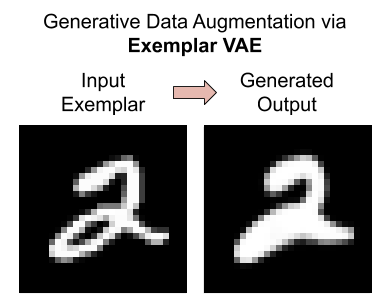## MotivationFirst Google image search result for ''A woman is staring at Monet's Water Lilies''.

Consider the problem of conditional image generation, given a natural language description of a scene such as:
''A woman is staring at Monet's Water Lilies''.
There are two general classes of methods for addressing this problem. One can resort to exemplar based methods, e.g., using web search engines to retrieve photographs with similar captions, and then editing the retrieved images to generate new ones. Alternatively, one can adopt parametric models such as deep neural networks optimized for text to image translation to synthesize new relevant scenes.

Exemplar based methods depend on large and diverse datasets of exemplars and relatively simple machine learning algorithms, such as Parzen window estimation and conditional random fields . They deliver impressive results on texture synthesis , image super resolution , and inpaiting , despite their simplicity. These techniques can accommodate web scale datasets with a improvement in sample quality as the dataset size increases, without the need for further optimization of model parameters. The success of exemplar based methods hinges on the distance metric used to build a local density model for each neighborhood. Further, while exemplar based methods excel in interpolation tasks, they often underperform their parametric counterparts in extrapolation.

Parametric generative models based on deep neural nets enable learning complex data distributions across myriad problem domains (e.g., ). Predominant models, such as Variational Autoencoders (VAEs), Normalizing Flows, and Generative Adversarial Networks (GANs) , adopt a decoder network to convert samples from a prior distribution, often a factored Gaussian, into samples from the target distribution. After the completion of training, these models discard the training data and generate new samples using decoder networks alone. Hence, the burden of generative modeling rests entirely on the parametric model. Further, with the availability of additional training data, these models require re-training or fine-tuning.

This work presents a probabilistic framework for exemplar based generative modeling using expressive neural nets. This framework combines the advantages of both exemplar based and parametric methods in a principled way and achieves superior results. We focus on simple unconditional generation tasks here, but the learning formulation and the methods developed are applicable to other applications including text to image translation and language modeling.

## Exemplar Generative Model

$$\renewcommand{\vec}{\boldsymbol{\mathbf{#1}}} \def\expected{\mathbb{E}} \def\t{T} \def\x{\vec{x}} \def\z{\vec{z}} \def\eg{{e.g.,} ~} \def\ie{{i.e.,} ~} \newcommand{\one}{{1}_{[#1]}}$$ We define an exemplar based generative model in terms of a dataset of $$N$$ exemplars, $$X \equiv \{\x_n\}_{n=1}^N$$, and a parametric transition distribution, $$\t_\theta(\x \mid \x')$$, which stochastically transforms an exemplar $$\x'$$ into a new observation $$\x$$. The log density of a data point $$x$$ under an exemplar based generative model $$\{X, \t_\theta\}$$ is expressed as \begin{equation} \log p(\x \mid X, \theta) ~=~ \log \sum\nolimits_{n=1}^N\frac{1}{N} \t_\theta(\x \mid \x_n) ~, \label{eq:exgen} \end{equation} where we assume the prior probability of selecting each exemplar is uniform. The transition distribution $$\t_\theta(\x \mid \x')$$ can be defined using any expressive parametric generative model, including VAEs, Normalizing Flow and auto-regressive models. Consistent with recent work , we find that simply maximizing the expected log marginal likelihood over the empirical training data, \begin{equation} O(\theta; X) ~=~ %\frac{1}{N} \sum\nolimits_{i=1}^N \log \sum\nolimits_{n=1}^N \frac{~1~}{N}\,\t_\theta(\x_i \mid \x_n) ~, \end{equation} to find the parameters of the transition distribution ($$\theta$$) results in massive overfitting. This is not surprising, since a flexible transition distribution can put all its probability mass on the reconstruction of each exemplar, $$\ie$$ $$p(\x \mid \x)$$, yielding high log-likelihood on training data but poor generalization. We propose two simple but effective regularization strategies to mitigate overfitting in exemplar based generative models:

### Leave-one-out during training

The generation of a given data point is expressed in terms of all exemplars except that point. The non-parametric nature of the generative model enables easy adoption of such a leave-one-out (LOO) objective during training, to optimize \begin{equation} O_1(\theta; X) ~=~ %\frac{1}{N} \sum_{i=1}^N \log \sum_{n=1}^N \frac{\one{i \neq n}}{N\!-\!1} \t_\theta(\x_i \mid \x_n)~, \end{equation} where $$\one{i \neq n} \in \{0, 1\}$$ is an indicator function taking the value of 1 if and only if $$i \neq n$$.

### Exemplar subsampling during training

In addition to LOO, we observe that explaining a training point using a subset of the remaining training exemplars improves generalization. To that end we use a hyper-parameter $$M$$ to define the exemplar subset size for the generative model. To generate $$\x_i$$ we draw $$M$$ exemplar indices, denoted $$\pi \equiv \{\pi_m\}_{m=1}^M$$, uniformly at random from subsets of $$\{1, \ldots, i-1, i+1, \ldots, N\}$$. Let $$\pi \sim \Pi^{N,i}_{M}$$ denote this sampling procedure with ($$N\!-\!1$$ choose $$M$$) possible subset outcomes. Combining LOO and exemplar subsampling, the objective takes the form \begin{equation} O_2(\theta; X) ~=~ %\frac{1}{N} \sum_{i=1}^N \mathop{\expected~~~~~~~~~}_{\pi\sim~\Pi^{N,i}_{M}} \log \sum_{m=1}^M %\one{i \neq \pi_m} \frac{1}{M} \t_\theta(\x_i \mid \x_{\pi_m}) ~. \label{eq:obj2} \end{equation}

## Exemplar VAE

We present theExemplar VAE as an instance of neural exemplar based generative models, in which the transition distribution in which $$\t(\x \mid \x')$$ is defined in terms of the encoder $$r_\phi$$ and the decoder $$p_\theta$$ of a VAE \begin{equation} \t(\x \mid \x') ~=~ \int_z r_\phi(\z \mid \x') \,p_\theta(\x \mid \z)\, d\z~. \end{equation} The Exemplar VAE assumes that, given $$\z$$, an observation $$\x$$ is conditionally independent from the associated exemplar $$\x'$$. This conditional independence assumption helps simplify the formulation, enabling efficient optimization. Marginalizing out the exemplar index $$n$$ and the latent variable $$\z$$, we derive an evidence lower bound (ELBO) on Exemplar VAE's log marginal likelihood for a single data point $$\x$$ as: \begin{eqnarray} &\log p(\x; X, \theta, \phi) \nonumber\\ &=~ \log \sum_{n=1}^N \frac{1}{N}\int_z {r_\phi(\z \mid \x_n) \,p_\theta(\x \mid \z)}\, d\z\\ &=~ \log \int_z {p_\theta(\x \mid \z)} \sum_{n=1}^N \frac{1}{N} r_\phi(\z \mid \x_n) \,d\z\\ &\ge \underbrace{\mathop{\expected}_{q_{\phi}(\z \mid \x)}\!\!\! \log p_{\theta}(\x\!\mid\!\z)}_{\mathrm{reconstruction}} - \!\!\underbrace{\mathop{\expected}_{q_{\phi}(\z \mid \x)} \log \frac{N\, q_\phi(\z \mid \x)}{\sum\nolimits_{n=1}^N r_\phi(\z \mid \x_n)}}_{\mathrm{KL~term}}. \label{eq:exVAE-ELBO} \end{eqnarray} The separation of the reconstruction and KL terms in summarizes the impact of the exemplars on the learning objective as a mixture prior distribution in the latent space, with each mixture component being defined using the latent encoding of one exemplar

## Experiments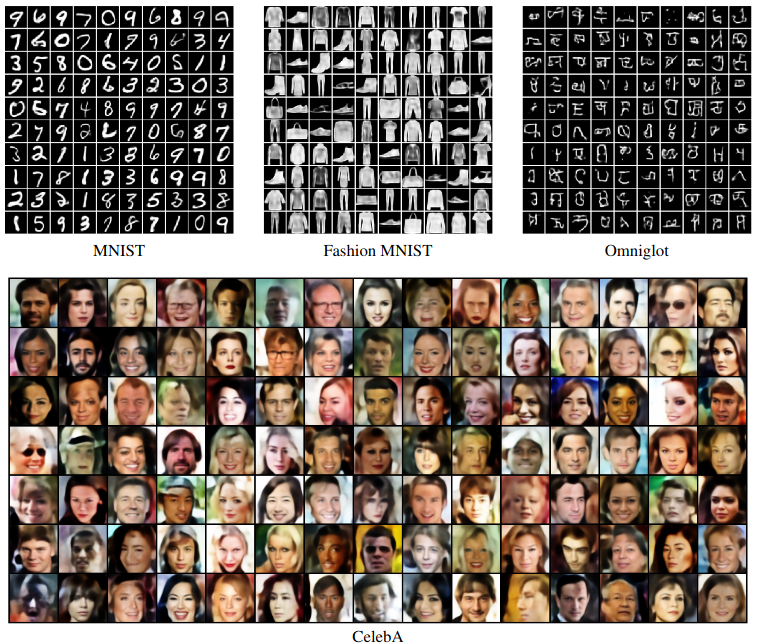To assess the effectiveness of Exemplar VAEs we conduct three sets of experiments, on density estimation, represenation learning, and unsupervised data augmentation.

### Density Estimation

We report density estimation with MNIST, Omniglot and Fashion MNIST, using three different architectures, namely VAE, HVAE and ConvHVAE . For each architecture we consider a Gaussian prior, the VampPrior, and an Exemplar based prior. For training VAE and HVAE we used the exact exemplar prior, but for ConvHVAE we used 10NN exemplars (see paper for the details).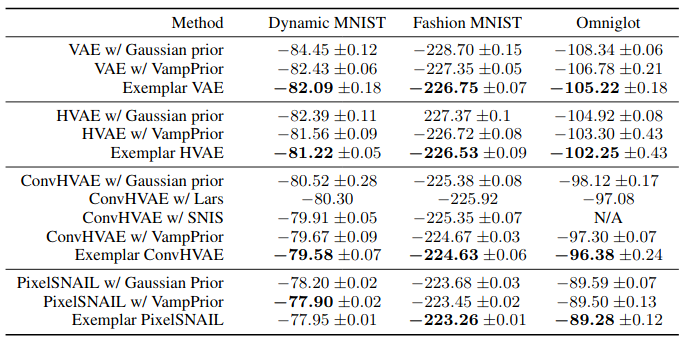Density estimation on dynamic MNIST, Fashion MNIST, and Omniglot for different methods and architectures. Log likelihoodlower bounds (in nats) averaged across 5 training runs are estimated using IWAE with 5000 samples.

### Representation Learning

We next explore the structure of the latent representation for Exemplar VAE. Images below show a t-SNE visualization of the latent representations of MNIST test data for the Exemaplar VAE and for VAE with a Gaussian prior.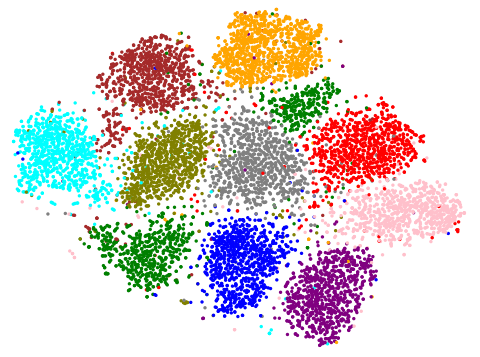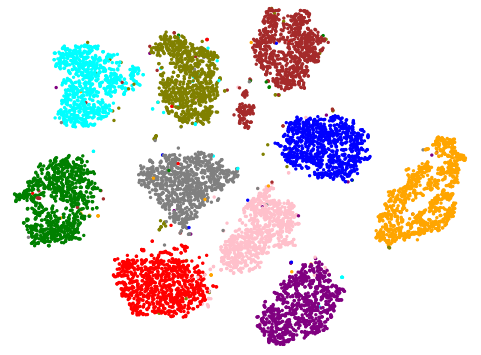Test points are colored by their digit label (No labels were used during training). We also use k-nearest neighbor (kNN) classification performance as a proxy for the representation quality. Exemplar VAE consistently outperforms other approaches.

### Generative Data Augmentation

We assess the effectiveness of the Exemplar VAE for generating augmented data to improve supervised learning. Recent generative models have achieved impressive sample quality and diversity, but they have seen limited success in improving discriminative models. In our experiments we use the training data points as exemplars and generate additional samples from the Exemplar VAE. Class labels of the exemplars are transferred to corresponding new images, and a combination of real and generated data is used for training.